# 关于Coronavirus数据简单的可视化分析(Plotly的一个例子)2020年5月13日07:20:55

1 14969字阅读49分53秒

## 数据集介绍

### Coronavirus的数据集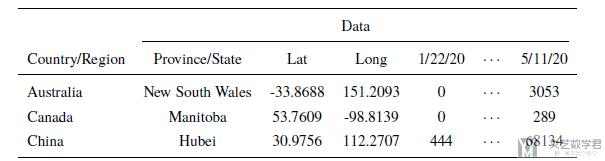### GDP, Population和Aging数据集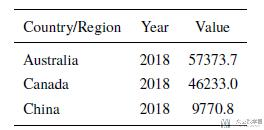## 数据处理

### 合并各省(州)数据

1. dict_groupby = {i:'sumfor i in confirm.columns.values[4:]}
2. dict_groupby['Lat'] = 'mean'
3. dict_groupby['Long'] = 'mean'
4. dict_groupby

1. {...
2.  '5/9/20': 'sum',
3.  '5/10/20': 'sum',
4.  '5/11/20': 'sum',
5.  'Lat': 'mean',
6.  'Long': 'mean'}

1. # confirmed cases
2. confirmCountry = confirm.groupby('Country/Region').agg(dict_groupby)
3. # confirmCountry.drop('Cruise Ship', inplace=True)

### 生成活跃人数数据集

1. # add active case: confirm-death-recovery
2. activeCountry = (confirmCountry - deathCountry - recoveredCountry)
3. activeCountry['Lat'] = -activeCountry.loc[:,'Lat'].values
4. activeCountry['Long'] = -activeCountry.loc[:,'Long'].values

### 新增变量-大洲与ISO3

1. import country_converter as coco

2. continent_name = coco.convert(names = list(confirmCountry.index.values), to='continent')
3. # 对每一个表格进行增加
4. confirmCountry['continent'] = continent_name
5. deathCountry['continent'] = continent_name
6. recoveredCountry['continent'] = continent_name
7. activeCountry['continent'] = continent_name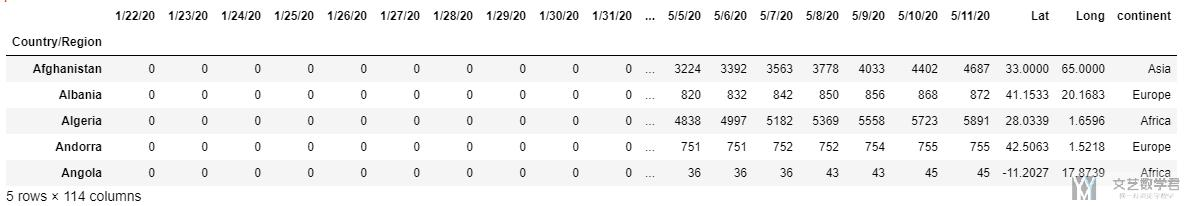2. country_name = confirmCountry.index.values
3. # 对每一个table进行增加国家名称
4. confirmCountry['Country/Region'] = country_name
5. deathCountry['Country/Region'] = country_name
6. recoveredCountry['Country/Region'] = country_name
7. activeCountry['Country/Region'] = country_name

2. confirmCountry['ISO3'] = confirmCountry.apply(lambda x : coco.convert(x['Country/Region'], to='ISO3', not_found=None), axis=1)
3. deathCountry['ISO3'] = deathCountry.apply(lambda x : coco.convert(x['Country/Region'], to='ISO3', not_found=None), axis=1)
4. recoveredCountry['ISO3'] = recoveredCountry.apply(lambda x : coco.convert(x['Country/Region'], to='ISO3', not_found=None), axis=1)
5. activeCountry['ISO3'] = activeCountry.apply(lambda x : coco.convert(x['Country/Region'], to='ISO3', not_found=None), axis=1)

### 数据合并-GPD,Aging,Population

1. # --------------------
2. # combine with GDP
3. # --------------------
5. # -------------------
6. # create new table
7. # ------------------
8. GDP_2018 = GDP_data.loc[:,['Country Code', '2018']] # 选取需要的字段
9. GDP_2018.rename(columns={'Country Code':'ISO3', '2018':'GDP_2018'}, inplace=True# 重命名
10. GDP_2018.dropna(subset=['GDP_2018'], inplace=True# 去除空值
11. # --------------
12. # start merge
13. # -------------
14. confirmCountry = pd.merge(confirmCountry, GDP_2018, on=['ISO3'])
15. deathCountry = pd.merge(deathCountry, GDP_2018, on=['ISO3'])
16. recoveredCountry = pd.merge(recoveredCountry, GDP_2018, on=['ISO3'])
17. activeCountry = pd.merge(activeCountry, GDP_2018, on=['ISO3'])

• 所属大洲, ISO3
• 这个国家的人均GDP
• 这个国家的老龄化情况这个国家的人口情况.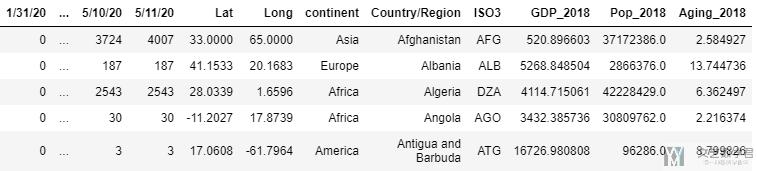### 数据格式的转换

1. # 1. confirmed cases
2. animationCountry = confirmCountry.copycopy()
3. animationCountry.reset_index(drop=True, inplace=True)
4. pd1 = pd.melt(animationCountry, id_vars=['Lat', 'Long', 'continent', 'Country/Region', 'ISO3', 'GDP_2018', 'Pop_2018', 'Aging_2018'], var_name = 'date', value_name='Confirmed')
5. # 2. death cases
6. animationCountry = deathCountry.copycopy()
7. animationCountry.reset_index(drop=True, inplace=True)
8. pd2 = pd.melt(animationCountry, id_vars=['Lat', 'Long', 'continent', 'Country/Region', 'ISO3', 'GDP_2018', 'Pop_2018', 'Aging_2018'], var_name = 'date', value_name='Death')
9. # 3. recovered cases
10. animationCountry = recoveredCountry.copycopy()
11. animationCountry.reset_index(drop=True, inplace=True)
12. pd3 = pd.melt(animationCountry, id_vars=['Lat', 'Long', 'continent', 'Country/Region', 'ISO3', 'GDP_2018', 'Pop_2018', 'Aging_2018'], var_name = 'date', value_name='Recovered')
13. # 4. active cases
14. animationCountry = activeCountry.copycopy()
15. animationCountry.reset_index(drop=True, inplace=True)
16. pd4 = pd.melt(animationCountry, id_vars=['Lat', 'Long', 'continent', 'Country/Region', 'ISO3', 'GDP_2018', 'Pop_2018', 'Aging_2018'], var_name = 'date', value_name='Active')

1. # product new dataset
2. data_frames = [pd1, pd2, pd3, pd4]
3. df_merged = functools.reduce(lambda left, right: pd.merge(left, right,on=['Lat', 'Long', 'continent', 'Country/Region', 'ISO3', 'GDP_2018', 'Pop_2018', 'Aging_2018', 'date']), data_frames)

1. # add two new variables
2. df_merged['deathRate'] = df_merged['Death']/df_merged['Confirmed']
3. df_merged['SickRate'] = df_merged['Confirmed']/df_merged['Pop_2018']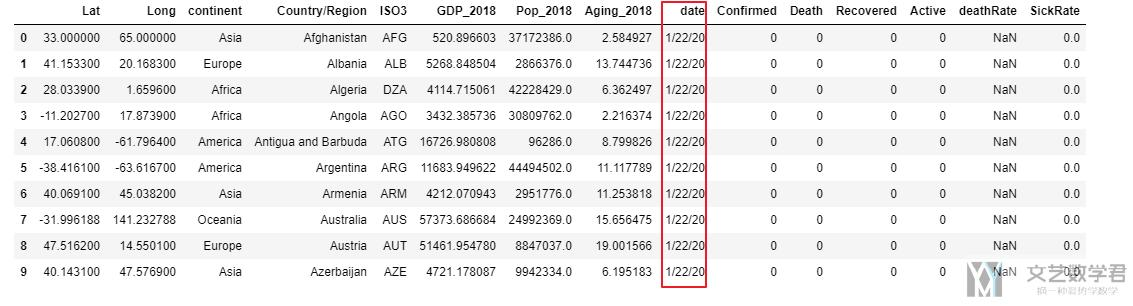## 关于Coronavirus数据的基础数据可视化

### 分析总体的变化趋势

1. matplotlib.style.use('default')
2. # Start
3. fig, ax1 = plt.subplots()
4. fig.set_size_inches(16, 8)
5. plt.set_cmap('RdBu')
6. # plt.xkcd()
7. # multiple line plot
8. pos = np.where(confirmCountry.columns.values==latest_date)+1 # 找出想画图画到的日期
9. x = confirmCountry.columns.values[:pos]
10. lw = 4
11. a, = ax1.plot(x, confirmCountry.sum().values[:pos], linewidth=lw, label='Confirmed Cases', marker='o') # confirm
12. b, = ax1.plot(x, activeCountry.sum().values[:pos], linewidth=lw, label='Active Cases', marker='o') # active
13. plt.legend(handles = [a,b], fontsize=15)
15. ax1.plot(['2/12/20', '2/12/20'], [0, 10000000], lw=3, linestyle='--', alpha=0.7)
16. ax1.plot(['3/12/20', '3/12/20'], [0, 10000000], lw=3, linestyle='--', alpha=0.7)
17. ax1.plot([latest_date, latest_date], [0, 10000000], lw=3, linestyle='--', alpha=0.7)
18. ax1.yaxis.set_tick_params(labelsize=15)
19. # ax1.set_xticks(x)
20. xticks = [i if i in ['1/22/20' ,'2/12/20', '3/12/20', latest_date] else '' for i in x] # x轴几个标记点
21. ax1.set_xticklabels(xticks, rotation=0, fontsize=15) # x轴设置trick
22. ax1.set_ylabel("Number of Cases (log)", fontsize='x-large')
23. ax1.set_xlabel('Date',  fontsize='x-large')
24. ax1.set_title('Worldwide Corona Virus Cases - Confirmed, Active (Line Chart)', fontsize='x-large')
25. plt.yscale('log')
26. # plt.ylabel('logy')
27. plt.show()

• 从1/22到2/12这段时间, active cases增长迅速
• 从2/12到3/12号这段时间, active cases出现了缓慢的下降.
• 但是从3/12开始, 这个数字又开始了快速的上升.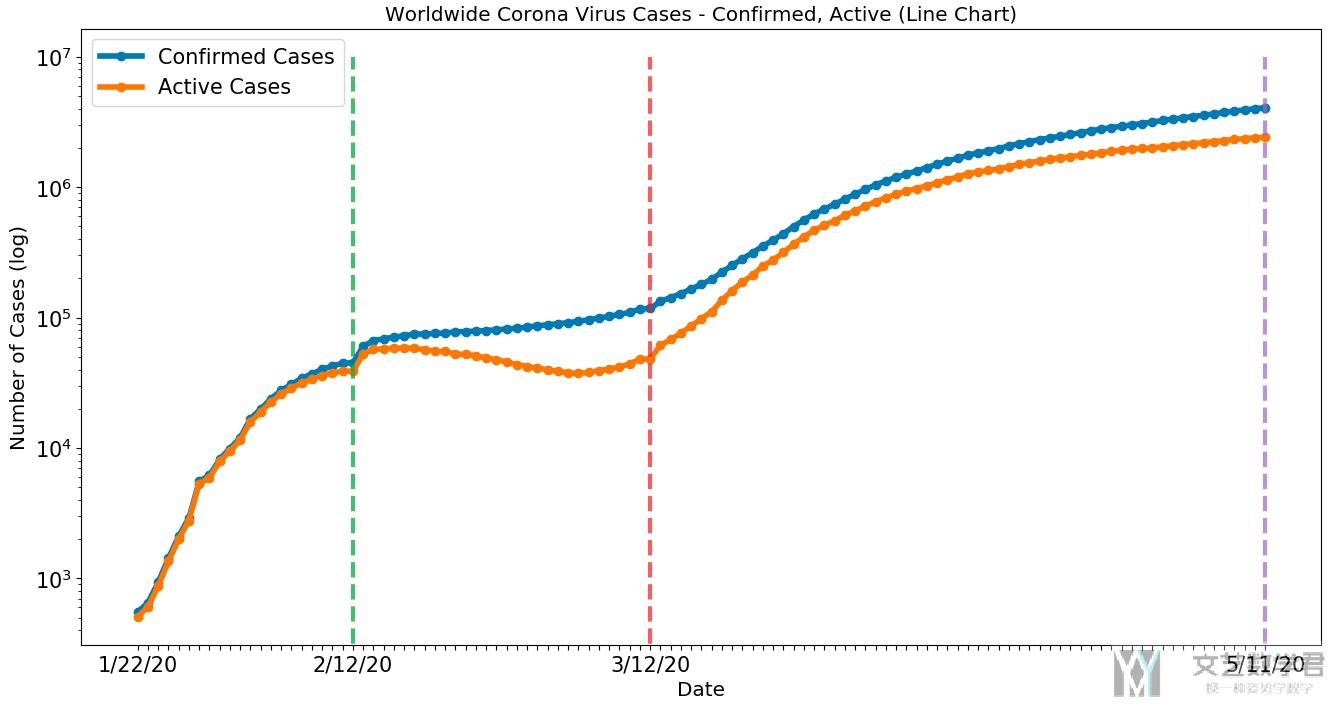### 对各个大洲进行分析

1. plotCountry = activeCountry.groupby('continent').sum()
2. plotCountry.drop(['Lat', 'Long', 'GDP_2018', 'Pop_2018', 'Aging_2018'], axis=1, inplace=True)
3. plotCountry['continent'] = plotCountry.index.values
4. matplotlib.style.use('default')
5. fig, ax1 = plt.subplots()
6. fig.set_size_inches(16, 8)
7. plt.set_cmap('RdBu')
8. x = plotCountry.columns.values[:-1] # 设置日期
9. lw = 2
10. for name in plotCountry.index.values:
11.     ax1.plot(x, plotCountry.loc[name].values[:-1], linewidth=lw, label=name, marker='o', markersize=2.5) # confirm
12. plt.legend(plotCountry.loc[:,'continent'])
13. ax1.plot(['2/12/20', '2/12/20'], [0, 1000000], lw=3, linestyle='--', alpha=0.7)
14. ax1.plot(['3/12/20', '3/12/20'], [0, 1000000], lw=3, linestyle='--', alpha=0.7)
15. ax1.plot([latest_date, latest_date], [0, 1000000], lw=3, linestyle='--', alpha=0.7)
16. ax1.yaxis.set_tick_params(labelsize=15)
17. # ax1.set_xticks(x)
18. xticks = [i if i in ['1/22/20', '2/12/20', '3/12/20', latest_date] else '' for i in x]
19. ax1.set_xticklabels(xticks, rotation=0, fontsize=15)
20. ax1.set_ylabel("Number of Cases (log)", fontsize='x-large')
21. ax1.set_xlabel('Date',  fontsize='x-large')
22. ax1.set_title('Active Corona Virus Cases in Each Continent - (Line Chart)', fontsize='x-large')
23. # set y scale
24. plt.yscale('log')
25. plt.show()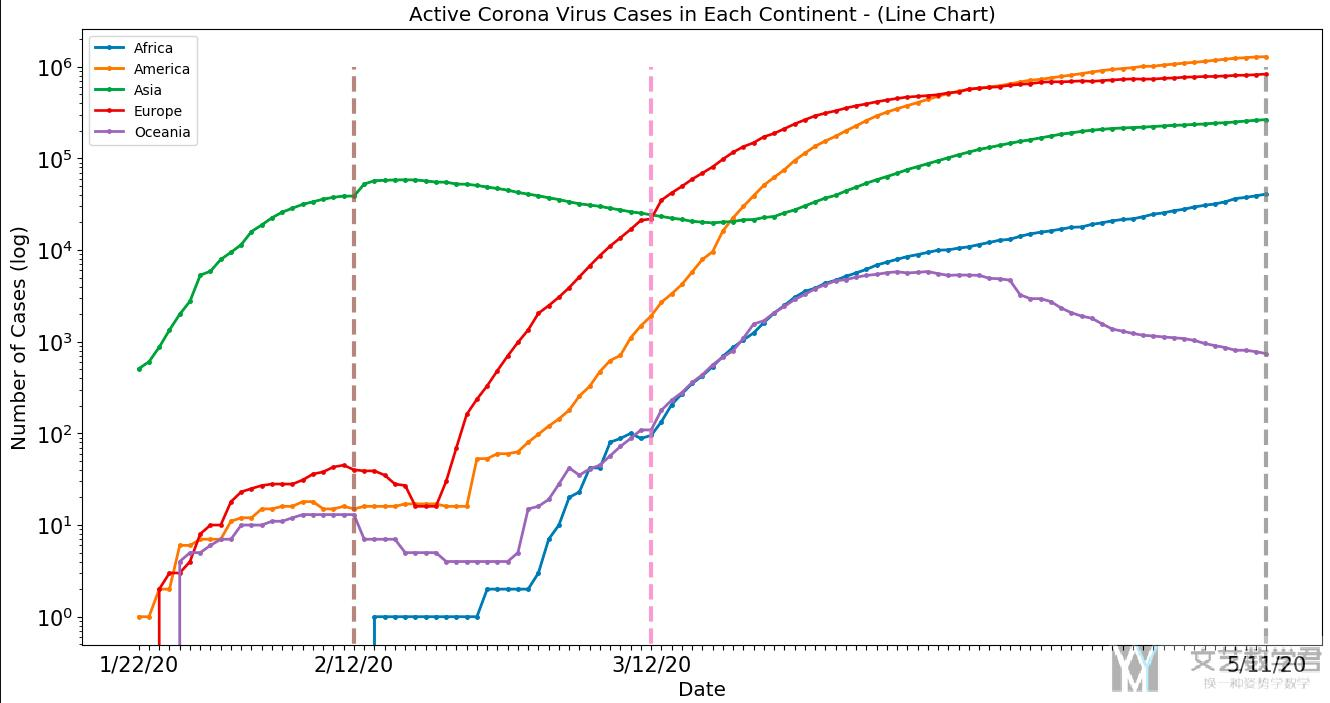• 绿色的曲线表示亚洲, 一开始亚洲开始上升, 2/12号到达高点之后感染人数开始下降.
• 欧洲, 美洲人数从2/12开始上升, 并且上升势头强劲.
• 亚洲在3/12之后感染人数又开始上升.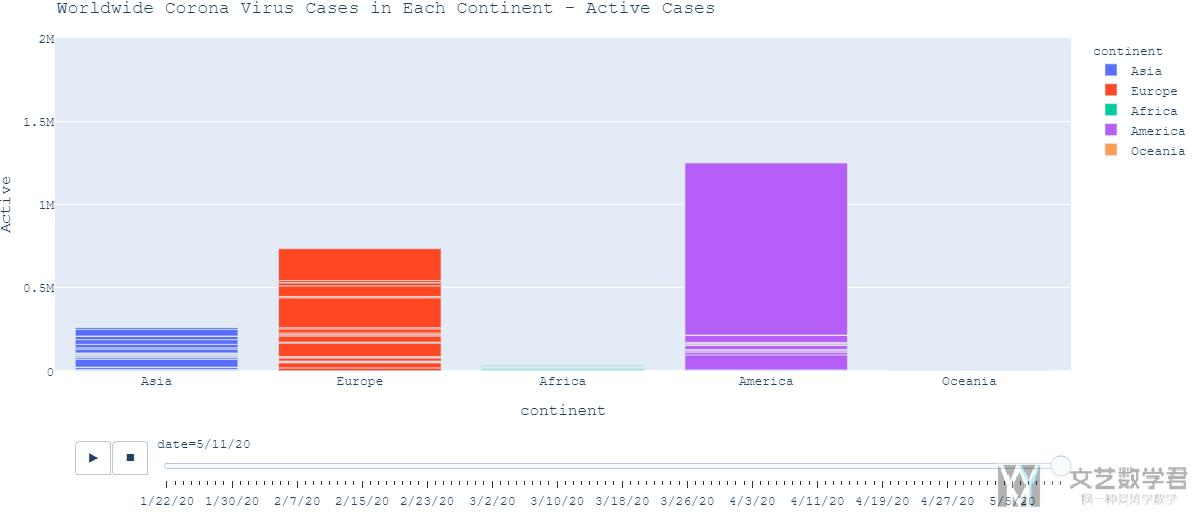### 对于各个国家的分析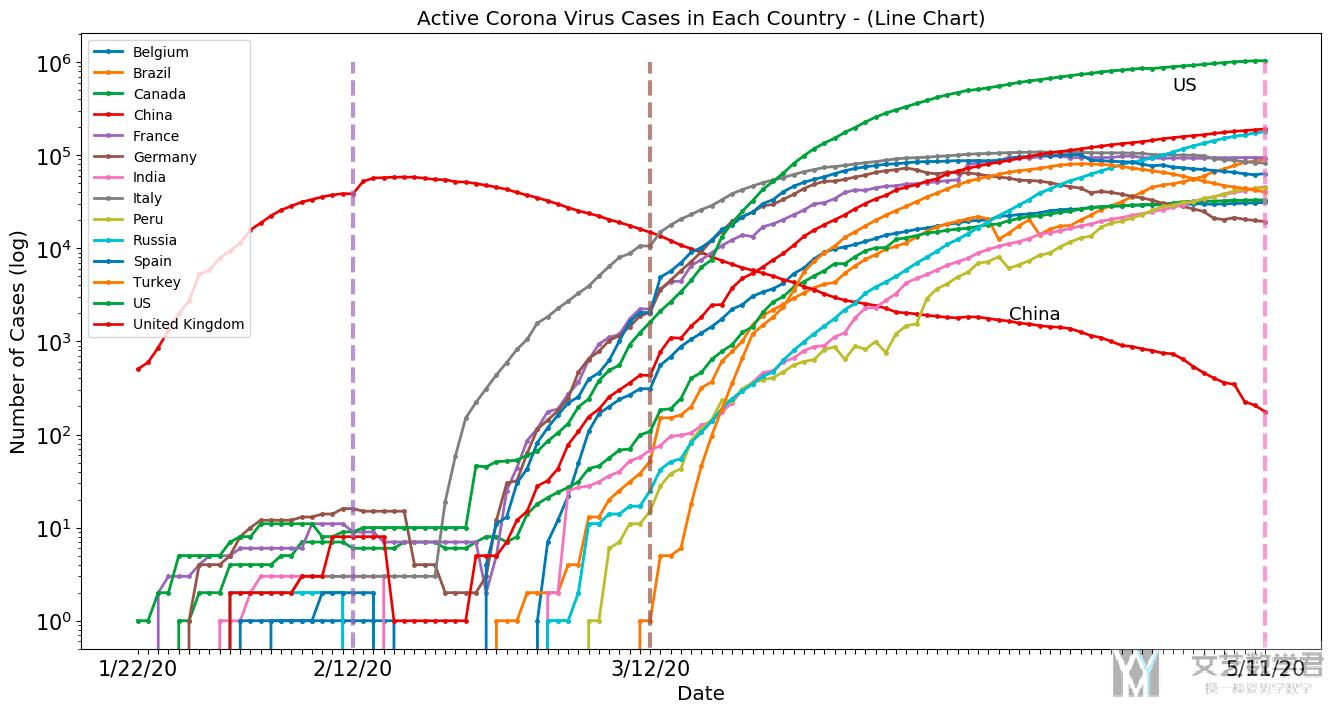• 一开始, 从亚洲的中国开始, 感染人数迅速上升, 并在2/12号到达顶点
• 接着从2/12号开始, 欧洲和美洲的国家感染人数开始快速上升, 特别是美国, 可以看到目前感染人数是最多的.
• 同时, 从3/12号开始, 亚洲其他国家, 例如印度和土耳其, 感染人数也在上升, 导致上面按大洲分析的时候后期亚洲的感染人数再次上升.

### 使用Sunburst Chart显示更多细节

1. # plot the active case in world wide
2. fig = px.sunburst(activeCountry, path=['continent', 'Country/Region'], values=latest_date,
3.                   color='continent',
4.                   color_continuous_scale='OrRd')
5. fig.update_layout(title='Worldwide Corona Virus Cases in Each Country and Continent - Active Cases',
6.                   font=dict(family="Courier New, monospace",
7.                             size=13)
8.                  )
9. fig.update_layout(margin={"r":0,"t":50,"l":0,"b":0})
10. fig.show()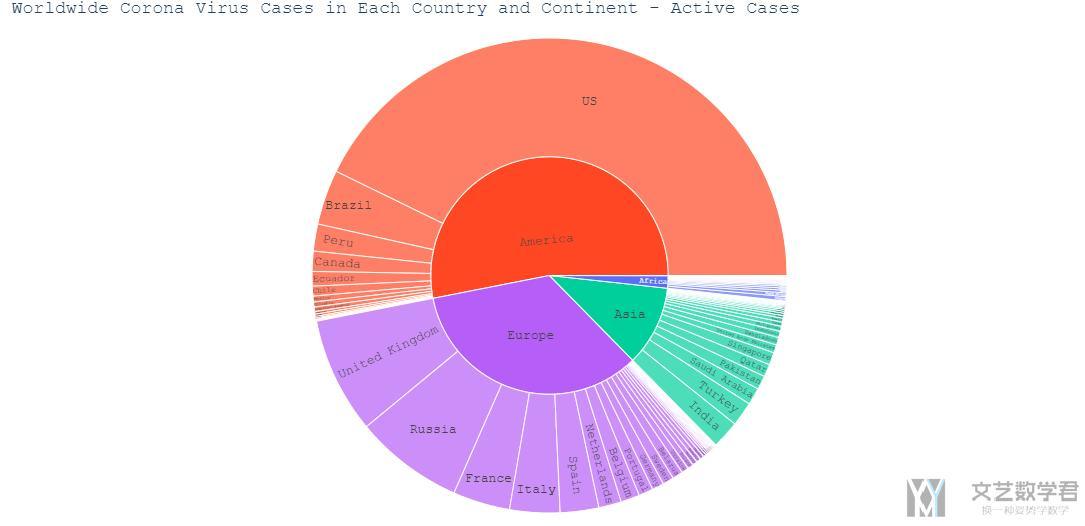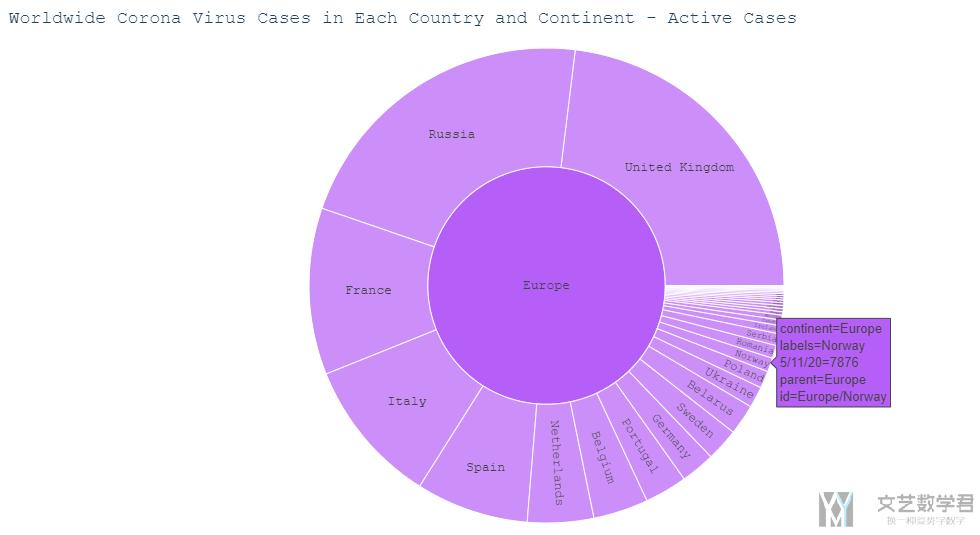### 结合地图进行可视化

1. fig = px.scatter_geo(df_merged[df_merged['date']=='5/11/20'],
2.                     locations = 'ISO3',
3.                     size='Active', size_max = 55, color="continent")
4. fig.update_layout(margin={"r":0,"t":50,"l":0,"b":0})
5. fig.update_layout(title='Worldwide Corona Virus Cases Time Lapse - Active',
6.                   font=dict(family="Courier New, monospace",size=13)
7.                  )
8. fig.show()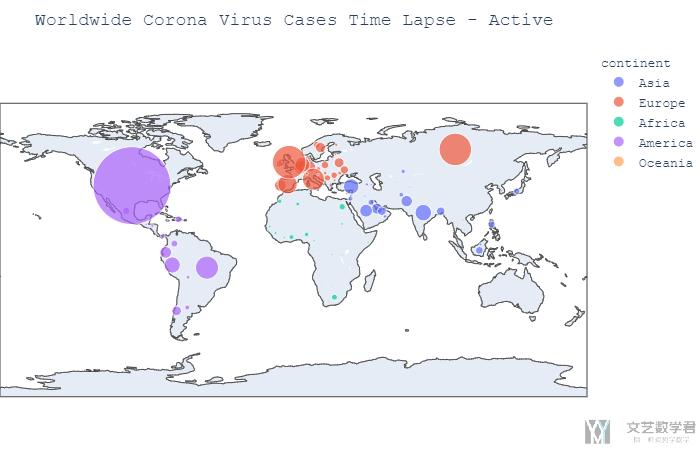1. fig = px.scatter_geo(df_merged,
2.                     locations = 'ISO3',
3.                     size='Active', size_max = 55,
4.                     animation_frame="date", animation_group='Country/Region',color="continent")
5. fig.update_layout(margin={"r":0,"t":50,"l":0,"b":0})
6. fig.update_layout(title='Worldwide Corona Virus Cases Time Lapse - Active',
7.                   font=dict(family="Courier New, monospace",size=13)
8.                  )
9. fig.show()

## 与其他数据集结合分析

### 直观分析

1. px.scatter(confirmCountry, x='GDP_2018', y='Aging_2018',
2.            color='continent', size=latest_date, size_max=60,
3.            hover_name="Country/Region", log_x=True)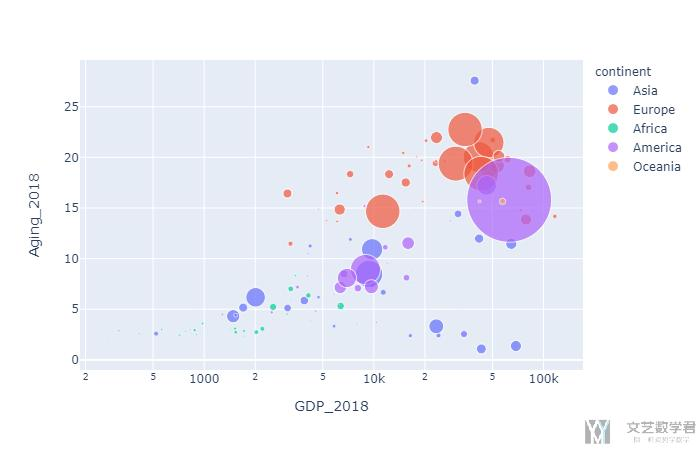### 计算相关系数

1. # Correlations for Dataset
2. correlationM = df_merged[df_merged['date']==latest_date][['GDP_2018','Pop_2018','Aging_2018','Confirmed', 'Death', 'Recovered', 'Active', 'deathRate', 'SickRate']]
3. correlationM.reset_index(drop=True, inplace=True)

1. # 绘制相关系数矩阵
2. plt.figure(figsize = (10,10))
3. ax = sns.heatmap(correlationM.corr(), annot=True, fmt='.1f', cmap="BuPu") # fmt表示保留的小数点
4. # 设置y轴的字体的大小
5. plt.yticks(rotation=0) # 让y轴的字进行旋转
6. # ax.yaxis.set_tick_params(labelsize=15)
7. plt.title('Correlations for Dataset', fontsize='xx-large')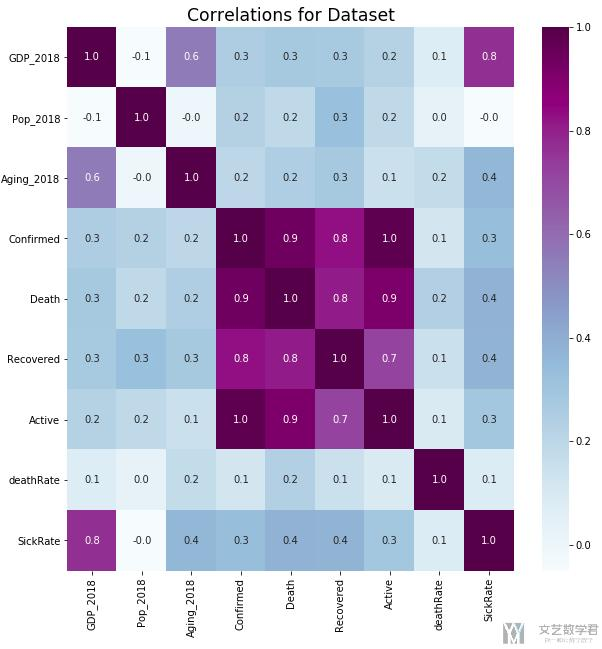### 简单的线性回归

1. px.scatter(df_merged[df_merged['date']==latest_date].dropna(subset=['deathRate','SickRate']),
2.            x='GDP_2018', y='SickRate',
3.            color='continent', size='Pop_2018', size_max=60,
4.            hover_name="Country/Region", log_x=True, log_y=True)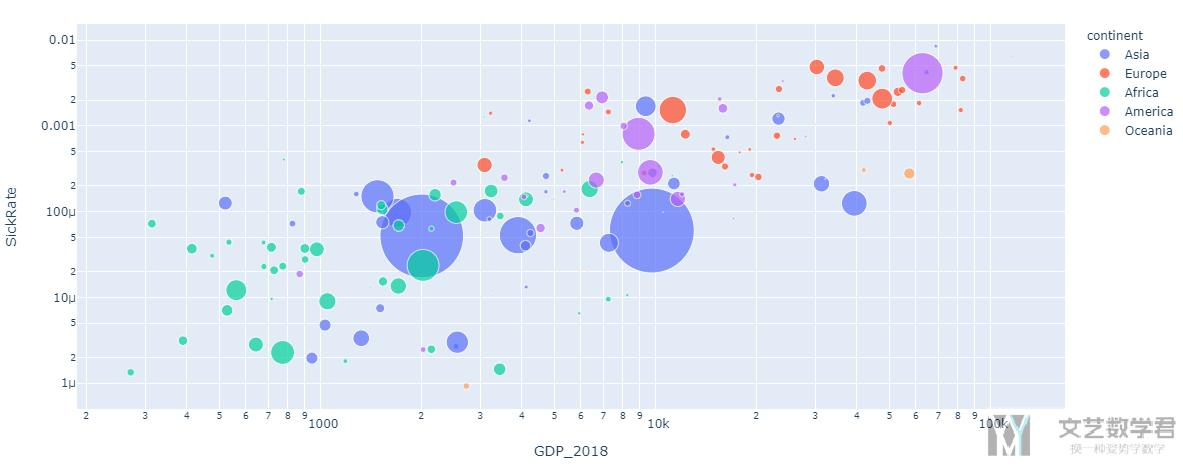• Each circle represent one country.
• The x-axis represents the GDP
• The y-axis represents the SickRate
• The size of the circle represents the number of confirmed cases.
• The color represent the continent.

1. x = df_merged[df_merged['date']==latest_date].dropna(subset=['deathRate','SickRate'])['GDP_2018'].values.reshape(-1,1)
2. y = df_merged[df_merged['date']==latest_date].dropna(subset=['deathRate','SickRate'])['SickRate'].values
3. weight = df_merged[df_merged['date']==latest_date].dropna(subset=['deathRate','SickRate'])['Pop_2018'].values
4. reg = LinearRegression().fit(np.log(x), np.log(y))
5. y_pre = reg.predict(np.log(x))
6. # get prediction
7. pred_dataframe = pd.DataFrame(x, columns=['x'])
8. pred_dataframe['y_pred'] = np.e**y_pre

1. r2_score(y_true=np.log(y), y_pred=y_pre)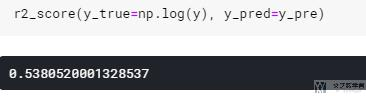1. fig = go.Figure()
2. fig = px.scatter(df_merged[df_merged['date']==latest_date].dropna(subset=['deathRate','SickRate']),
3.            x='GDP_2018', y='SickRate',
4.            color='continent', size='Pop_2018', size_max=60,
5.            hover_name="Country/Region", log_x=True, log_y=True)
7.                          y=pred_dataframe['y_pred'],
8.                          mode='lines',
9.                          marker_color='rgba(152, 0, 0, .8)',
10.                          name='Regression'))
11. fig.update_layout(xaxis_type="log")
12. fig.show()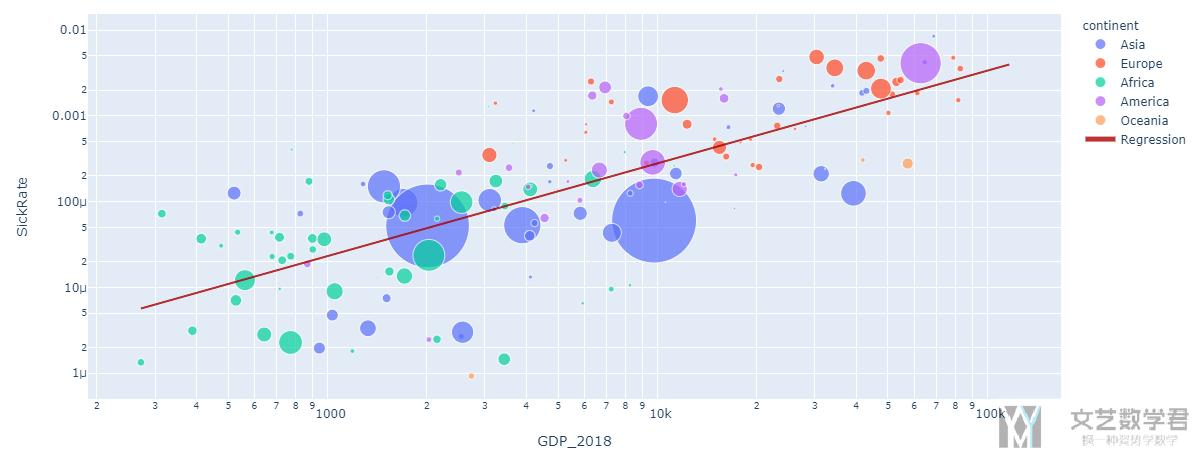• 微信公众号
• 关注微信公众号
•• QQ群
• 我们的QQ群号
•• 本文由 发表于 2020年5月13日07:20:55
• 转载请务必保留本文链接：https://mathpretty.com/12198.html